Mathematics and Computer Science
Volume 1, Issue 4, November 2016, Pages: 82-85

The Gray Segmentation of Color Image Based on Level Set Method

Wang Wenjie, Feng Jianhu

College of Science, Chang’an University, Xi’an, China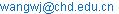(Wang Wenjie)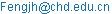(Feng Jianhu)

Wang WenJie, Feng JianHu. The Gray Segmentation of Color Image Based on Level Set Method. Mathematics and Computer Science. Vol. 1, No. 4, 2016, pp. 82-85. doi: 10.11648/j.mcs.20160104.12

Received: July 4, 2016; Accepted: July 20, 2016; Published: October 18, 2016

Abstract: Firstly, the flaw of usual colored image to gray was discussed. And a new method of colored image to gray based on gradient domain was proposed. Secondly, the gray image was segmented using the level set method. Finally, it was compared the method of this paper with the traditional level set method. The numerical examples shown that the new method is stable and it can deal with the case of the topology changeand it can advance the accuracy of image segmentation.

Keywords: Colored Image to Gray Image, Level Set Method, Gradient Domain, Image Segmentation

Contents

1. Introduction

At present, although the color image has been widely used, in many cases, the gray image is still needed to use and need to convert color image to gray image. Each pixel gray value of the gray image is generally obtained by the simple weighted sum to the red, green, blue three color space brightness for the traditional color image gray method. However, it is difficult to distinguish for the region of the color image which the brightness is same or similar and the color is different based on the traditional method of gray scale. Therefore, our goal is not only to grayscale images as accurate as possible to reflect the brightness of the color image, but also in the gray image as much as possible to retain the details of the color image can be distinguished.

Image segmentation, as an image preprocessing method, has attracted more and more attention. People have put forward a lot of effective and robust segmentation algorithms [1-5], and have been applied to many fields of science and engineering. And in those, the most inspiring should be the level set method which is proposed by Osher and Sathian  in 1998. This method overcome the weakness of other algorithms, such as the sensitivity of segmentation results to the initial position, the weakness of the topological adaptability and the difficultness to separate structure more complex objects and so on.

In this paper, we proposed a method of color image gray transformation based on gradient domain, and discussed the basic idea of the level set method and used the level set method for image segmentation.

2. Color Image Gray

Firstly, the color image is transformed into LCrCb space, and then the gradient of each component is calculated. If the gradient is larger, then keep its gradient unchanged; if the gradient is small, and the color difference component Cr or Cb gradient of the corresponding position of is larger, then keep the gradient direction unchanged, increase its gradient value. Finally, the gray image is obtained by solving the Poisson equation.

Letis the luminance component of the LCrCb spatial,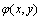is recorded as the gradient magnification function, and the modified gradient is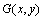, then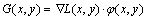(1)

According to the method of literature , searching the function space of two-dimension, make the gradient of gray image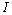close to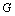in the least square difference. That is to minimize the integral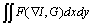(2)

In which,.

According to the variational principle, the functionis minimized (2) in the integral, must meet the Euler-Lagrange equation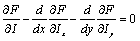(3)

Substitution and simplification, can obtain Poisson's equation: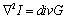(4)

In which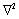is the Laplasse operator,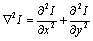;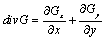is the divergence of the gradient. The gray image is obtained by solving this Poisson equation.

The following is the definition of gradient amplification function. Firstly, calculates the average values of the luminance gradient and the chroma gradient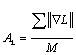,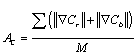.

In which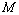is the total number of pixels. For the brightness gradient value is smaller than its average value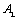and the sum of the two chroma gradient is greater than the average value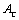of the pixel, the gradient is enlarged, the function is defined as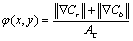. Thus, the greater of the chroma gradient, the larger of the magnification; the other conditions remain unchanged, that is,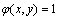.

3. Level Set Method for Image Segmentation

Let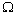is a single connected region,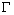is a moving open subset, whose boundary is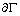, the internal region of the open subsetis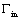, that is,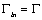. Suppose that the intensity of the color image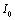can be divided into two different values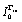and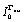, whereis the intensity of the target image, and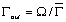.

According to the literature , we can get the following form of the variational problem: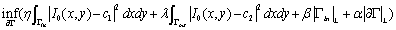(5)

In which the constant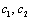is the average intensity image in the region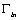and in the medium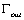of the original corresponding. Thus, when the energy functional (5) reaches minimum, the boundaryof the moving open subsetreaches the edge of the target image.

Then, the corresponding Euler-Lagrange equation of the variational problem (5) is:(6)

In order to maintain the smooth of the zero level set, and to avoid the appearance of small and isolated regions in the final segmentation results, we need to add the length penalty term in the regularization term, which is related to the length of the evolution curve. Let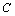is a smooth closed planar curve: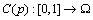, the parameter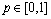, so the length of the curve can be expressed: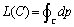. The curvecan be substituted for the zero level set of the level set function, and the length of the curvecan be expressed as: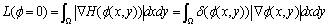(7)

In which,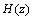and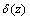are Heidegger's function and Dirac function respondingly. The effect of the length penalty term is that: it has a binding effect to the change of the length of the curve making the evolution curve keep as short as possible in minimum of the total energy functional. At this time, the image can be divided into two parts: foreground object and background. The energy functional will have a conversion process between the mean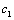/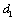of the inside of the curve and the mean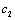/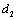of the outside of the curve. In many cases, The shock will occur for the level set function after the evolution of a period of time, and gradually lose the characteristics of smooth and distance functions. As the evolution continues, the error will continue to accumulate and cause the final curve to deviate from the true target edge. In order to avoid the emergence of these problems, the level set function is constructed as the sign distance function in the general level set method. At the moment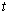, this process can be written as :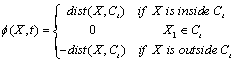(8)

in which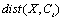represents the shortest Euclidean distance of the point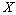and the evoluted curve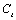.

In order to guarantee the final segmentation accuracy, it is very necessary to use the level set function to maintain the approximate sign distance function in the evolution process, especially in the neighborhood of the zero level set. And the most direct way to re initialization is to extract the evolution curve (zero level set), and then calculate the shortest Euclidean distance of all points on the image and the level set. this is a very time consuming process. In order to reduce the computation of reinitialization, Sussman et al (1999) proposed a distance function iteration method, which can be realized by solving the following partial differential equations with the time variation of the level set function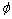: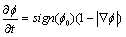, in which,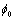is the level set function which needs to be initialized,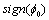is a symbolic function. When the partial differential equation is stable, the level set functionwill be a distance function with the same level set for.

Reinitialization is widely used as a numerical technique in the level set method, which can ensure the stability of the curve evolution and the accuracy of the evolution. However, it is clear that the reinitialization also appear the case of in consistent to the theory and the numerical realization for the level set method, and may make the zero level set from the original location. In addition, whether it is to take the distance function iteration method or short distance equation method, the calculation of the larger amount is also a problem, and when and how to reinitialization is a problem too.

In order to accelerate the iterative process of solving the Euler-Lagrange equation (6), in this paper, we replace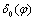for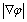. So the equation (6) can be rewritten as:(9)

Finally, we use the numerical method to solve the equation (9) in the literature , and get the result of the segmentation of the color image after the gray scale.

4. Numerical Results

We use a writing brush and sunflower pictures on the algorithm proposed in this paper. In Fig. 1 and Fig. 2, (a) express the original image; (b) is the segmentation image obtained using the traditional level set method and (c) express the segmentation image obtained by the method of this paper. As can be seen from the graph, when using the traditional level set method, Fig. 1 (b) and Fig. 2 (b) "" word and petals of several sharp been cut off and it can not located to the complex objects. Using the method described in this paper can accurately outline the "news" word and the outer contour of the petals, such as Fig. 1 (c) and Fig. 2 (c).Fig. 1(a). The original image.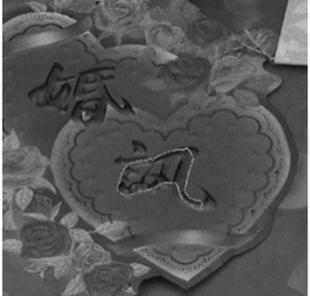Fig. 1(b). The traditional level set method.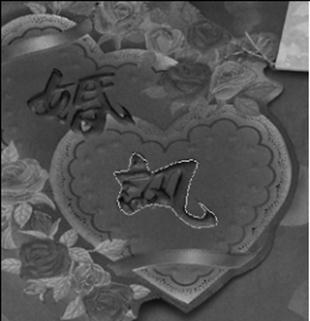Fig. 1(c). The method of in this paper.Fig. 2(a). The original image.Fig. 2(b). The traditional level set method.Fig. 2(c). The method of in this paper.

5. Conclusions

Through the numerical examples, we can see that this method can not only improve the accuracy of color image segmentation, but also can adapt to the change of topology structure.

References

1. Wang Xincheng. Advanced image processing technology [M]. Beijing: China Science and Technology Press, 2000(in Chinese).
2. Luo Xiping, Tian Jie. An overview of image segmentation methods [J]. Pattern recognition and artificial intelligence, 1999,9 (3); 300-312(in Chinese).
3. Xiao Chaoyun, Zhu Weixing. Threshold segmentation algorithm based on Otsu criterion and image entropy [J]. Computer Engineering, 2007,33 (14):188-190(in Chinese).
4. Chan T F,Vese L A. Active Contours Without Edges[J]. IEEE Trans on Image Processing,2001,10(2):266-277.
5. You Jianjie, Zhou Zeming,Pheng Ann Henget al. Simulated Annealing Based Simplified Snakes for Weak Edge Medical Image Segmention[J]. Journal of Image and Graphics,2004,9(1):11-17.
6. Osher S., Sethian J.A. Front propagating withcurvature-Dependent Speed: Algorithm based on Hamilton-Jacobin Formulation[J]. Computational physics,1988 (79):12-49.
7. Fattal R., Lischinski D., Werman M., Gradient Domain High Dynamic Range Compression[J], Proc, ACM Siggraph 2002,21(3),249-256.
8. Zhou Bingfeng, Zhang Weixiang. A method based on gradient domain for color image to gray level image, image technology, 2007 (3), 20-22(in Chinese).
9. Chan T., Vese L., Active contours without edges[J]. IEEE Transaction ImageProcessing,2001,10(2):266-277.
10. Wang Xiaofeng, Doctoral thesis,Univercity of Science and Technology of China,2009,55-57(in Chinese)
11. Wang Wenjie, Feng Jianhu. Image segmentation based on variational Set Level method, computer engineering and applications, 2006 (18):68-18(in Chinese).

Biography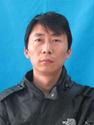Wenjie Wang, lecturer. Research direction: Image processing based on partial differential equations. Feng Jianhu, professor. The equation of hyperbolic conservationlaws.
 Contents 1. 2. 3. 4. 5.
Article ToolsAbstractPDF(1237K)